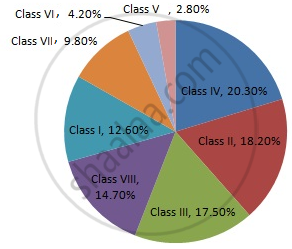Share

# Draw a Pie-diagram Representing the Relative Frequencies (Expressed as Percentage) of the Eight Classes as Given Below: 12.6, 18.2, 17.5, 20.3, 2.8, 4.2, 9.8, 14.7 - Mathematics

Course
ConceptCircle Graph Or Pie Chart - Drawing Pie Charts

#### Question

Draw a pie-diagram representing the relative frequencies (expressed as percentage) of the eight classes as given below:
12.6, 18.2, 17.5, 20.3, 2.8, 4.2, 9.8, 14.7

#### Solution

We know:
Central angle of a component = (component value/sum of component values x 360)
Here, total amount = 100.1%
Thus, central angle for each component can be calculated as follows:

 Item Amount (in %) Sector angle Class I 12.6 12.6/100.1 x 360 = 45.3 Class II 18.2 18.2/100.1 x 360 = 65.5 Class III 17.5 17.5/100.1 x 360 = 62.9 Class IV 20.3 20.3/100.1 x 360 = 73 Class V 2.8 2.8/100.1 x 360 = 10.1 Class VI 4.2 4.2/100.1 x 360 = 15.1 Class VII 9.8 9.8/100.1 x 360 = 35.2 Class VIII 14.7 14.7/100.1 x 360 = 52.9

Total = 100.1%

Now, the pie chat representing the given data can be constructed by following the steps below:
Step 1 : Draw circle of an appropriate radius.
Step 2 :  Draw a vertical radius of the circle drawn in step 1
Step 3 : Choose the largest central angle. Here the largest central angle is 73o. Draw a sector with the central angle 73o in such a way that one of its radii coincides with the radius drawn in step 2 and another radius is in its counter clockwise direction.
Step 4 : Construct other sectors representing the other items in the clockwise sense in descending order of magnitudes of their central angles.
​Step 5 : Shade the sectors with different colours and label them, as shown as in the figure below.​​Is there an error in this question or solution?

#### APPEARS IN

RD Sharma Solution for Mathematics for Class 8 by R D Sharma (2019-2020 Session) (2017 to Current)
Chapter 25: Data Handling-III (Pictorial Representation of Data as Pie Charts or Circle Graphs)
Ex. 25.1 | Q: 15 | Page no. 14

#### Video TutorialsVIEW ALL 

Solution Draw a Pie-diagram Representing the Relative Frequencies (Expressed as Percentage) of the Eight Classes as Given Below: 12.6, 18.2, 17.5, 20.3, 2.8, 4.2, 9.8, 14.7 Concept: Circle Graph Or Pie Chart - Drawing Pie Charts.
S